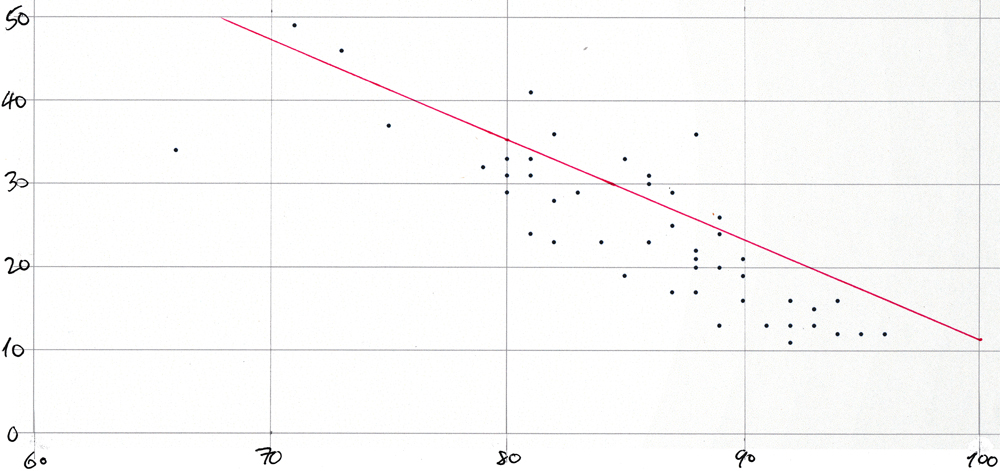# Average COVID-like symptoms vs average mask-wearing by state scatter plot for 21-Oct-2020

## Scatter-plot for 21-Oct-2020

The dots on this plot represent "mask_wearing_pct" on the x-axis with "symptoms_pct" on the y-axis from this query:

``````select
round(symptoms_pct)      as "% with symptoms",
state
from covidcast_fb_survey_results_v
where survey_date = to_date('2020-10-21', 'yyyy-mm-dd')
order by 1;
``````

The plot would be too cluttered if each of the 51 points were labeled with its two-letter state abbreviation.

The plot was created simply by pasting a comma-separated list of "mask_wearing_pct"-"symptoms_pct" pairs into a spreadsheet and by using the app's built-in functionality to create a scatter plot from such pairs values. The values were produced with this query:

``````select
from covidcast_fb_survey_results_v
where survey_date = to_date('2020-10-21', 'yyyy-mm-dd')
order by 1;
``````

Then the plot was printed and the line was drawn in by hand using the slope and y-axis intercept from this query:

``````with a as (
select
max(survey_date)                               as survey_date,
from covidcast_fb_survey_results_v
where survey_date = to_date('2020-10-21', 'yyyy-mm-dd'))
select
to_char(survey_date,      'mm/dd')  as survey_date,
to_char(s,  '90.9')                 as s,
to_char(i,  '990.9')                as i
from a;
``````

This is the result:

`````` survey_date |   s   |   i
-------------+-------+--------
10/21       |  -1.2 |  131.4
``````

And here is the plot:## Scatter-plot for synthetic data

For comparison, the same technique was used to create a scatter-plot and to draw in the best-fit straight line using synthetic data by running the procedure "populate_t()" described in the section Create the test table within the section that introduces the built-in aggregate functions for linear regression analysis.

``````create table t(
k      int primary key,
x      double precision,
y      double precision,
delta  double precision);

create procedure populate_t(
no_of_rows  in int,
slope       in double precision,
intercept   in double precision,
mean        in double precision,
stddev      in double precision)
language plpgsql
as \$body\$
...
``````

This code, and the remaining code below, needed to make the scatter-plot for synthetic data is included in `synthetic-data.sql`.

It uses the function `normal_rand()`, brought by the tablefunc extension to add pseudorandomly generated normally distributed noise the y-axis values produced by the "y = m*x + c" formula for the straight line.

It was then invoked like this:

``````call populate_t(
no_of_rows  => 100,
mean        => 0.0,
stddev      => 5.0,
slope       => -1.2,
intercept   => 131.4);
``````

using the values for slope and intercept from the regression analysis of the COVIDcast data for 21-Oct-2020 and by choosing a value for the "stddev" actual argument arbitrarily.

The comma-separated pairs for the spreadsheet were produced by this query:

``````select
round(x)::text||','||round(y + delta)::text
from t
where
x > 60        and
x < 95        and
x is not null and
y is not null
order by x;
``````

And the values for the slope and y-axis intercept were produced by this query:

``````with a as (
select
regr_r2       ((y + delta), x) as r2,
regr_slope    ((y + delta), x) as s,
regr_intercept((y + delta), x) as i
from t)
select
to_char(r2,  '0.99')                as r2,
to_char(s,  '90.9')                 as s,
to_char(i,  '990.9')                as i
from a;
``````

This is the result:

``````  r2   |   s   |   i
-------+-------+--------
0.98 |  -1.2 |  130.8
``````

The emergent values for the slope and intercept are very close to the values (-1.2 and 131.4) that were used for the invocation of "populate_t()".

Here is the resulting plot:

Note: The `normal_rand()` function produces a different set of pseudorandomly distributed values each time that `synthetic-data.sql` is run. But the overall shape of the scatter-plot will remain the same.